Courses

# Test: Motion in a Straight Line- 2

## 30 Questions MCQ Test Physics Class 11 | Test: Motion in a Straight Line- 2

Description
This mock test of Test: Motion in a Straight Line- 2 for JEE helps you for every JEE entrance exam. This contains 30 Multiple Choice Questions for JEE Test: Motion in a Straight Line- 2 (mcq) to study with solutions a complete question bank. The solved questions answers in this Test: Motion in a Straight Line- 2 quiz give you a good mix of easy questions and tough questions. JEE students definitely take this Test: Motion in a Straight Line- 2 exercise for a better result in the exam. You can find other Test: Motion in a Straight Line- 2 extra questions, long questions & short questions for JEE on EduRev as well by searching above.
QUESTION: 1

### A particle is projected with a velocity v, so that its range on a horizontal plane is twice the greatest height attained. If g is acceleration due to gravity, then its range is

Solution: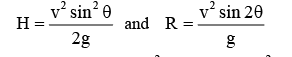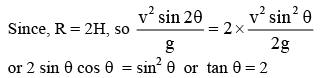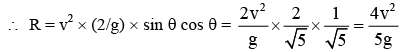QUESTION: 2

### A particle is fired with velocity v making an angle q with the horizontal. What is the magnitude of change in velocity when it is at the highest point?

Solution:

velocity at the highest point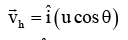velocity at the starting point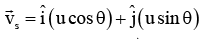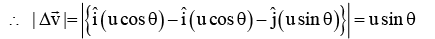QUESTION: 3

### A person can throw a stone to a maximum distance of 100 m. The greatest height to which he can throw the stone is

Solution:

Maximum height is given by H =2gu2sin2θ​

Range is given as R =gu2sin2θ​=100m(given)

Maximum height is attained at 45 degree launch, so θ=45

u2=1000 and Maximum height is 25 m by formula of H.

QUESTION: 4

The ceiling of a hall is 40 m high. For maximum horizontal distance, the angle at which the ball may be thrown with a speed of 56 m/s without hitting the ceiling of the hall is

Solution: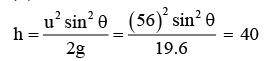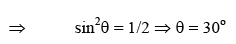QUESTION: 5

A bomb is dropped from an aeroplane when it is directly above a target at a height of 1254.4 m. The aeroplane is going horizontally with a speed of 150 m/s. The distance by which it will miss the target is

Solution:

using the formula:
D = u√2H/g
Where D is distance, u is initial velocity, H is height and g is acceleration due to gravity.
Putting the values,
D= 150√2×1254.4/9.8
D= 150√2508.8/9.8
D= 150√256
D= 150×16
D= 2400m
D= 2.4 km

QUESTION: 6

The  range R of projectile is same when its maximum height are h1 and h2. What is the relation between R, h1 and h2?

Solution:

Range is same for angles of projection θ and 900 - θ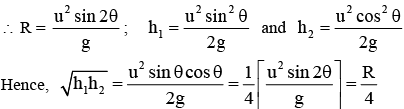QUESTION: 7

The friction of the air causes a vertical retarding equal to 10% of the acceleration due to gravity. The time taken to return to the ground from the maximum height

Solution: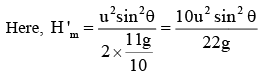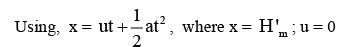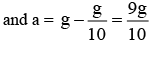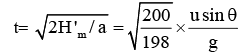It is almost equal to the time of fall in the absence of friction.

QUESTION: 8

A ball rolls off the top of a staircase with a horizontal velocity u m/s. If the steps are h meter high and b meter wide, the ball will hit the edge of the nth step, if

Solution:

If the ball hits the nth step, then horizontal distance traversed = nb

Vertical distance traversed = nh

Here, velocity along horizontal direction = u

Velocity along vertical direction = 0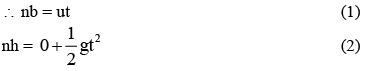from equation one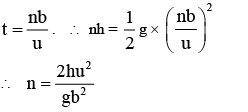QUESTION: 9

A projectile is fired from level ground at an angle θ above the horizontal. The elevation angle of the highest point as seen from the launch point is related to θ by the relation

Solution:

It is clear from the adjoining figure,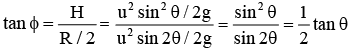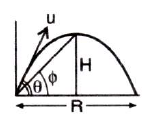QUESTION: 10

A projectile has a time of flight T and range R. For a given angle of projection if the time of flight is doubled, then what happens to the range?

Solution: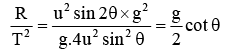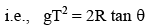If T is doubled, then R becomes 4 times.

QUESTION: 11

A ball is projected from the ground at an angle q with the horizontal. After 1 second it is moving at angle 45o with the horizontal and after 2 seconds it is moving horizontally. What is the velocity of projection of the ball ?

Solution:

Suppose the angle made by the instantaneous velocity with the horizontal be α. then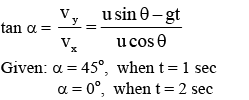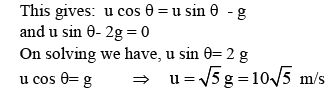QUESTION: 12

hree particles A, B and C are projected from the same point with the same initial speeds making angles 30o, 45o and 60o respectively with the horizontal. Which of the following statements is correct?

Solution:

when a projectile is projected at an angle θ or at angle ( 90o - θ ) with the horizontal, the horizontal range remains the same. The horizontal range is maximum  when the angle of projection is 45o

QUESTION: 13

The speed of a projectile when it is at its greatest height, is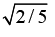times its speed at half the maximum height. The angle of projection is

Solution:

Let θ be the angle of projection and u its initial speed. Then maximum height will be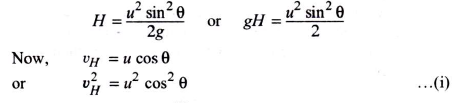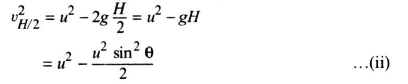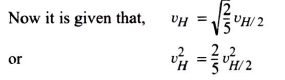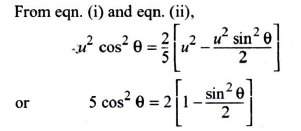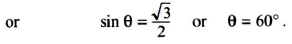QUESTION: 14

A particle is projected from the ground with an initial speed of v at an angle q with horizontal. The average velocity of the particle between its point of projection and highest point of trajectory is

Solution:

Average velocity = Displacement/Time

Here,  H = maximum height =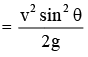R = range =nd T = time of flight =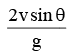QUESTION: 15

A particle is projected with a certain velocity at an angle a above the horizontal from the foot of an inclined plane of inclination 30o. If the particle strikes the plane normally then a is equal to

Solution: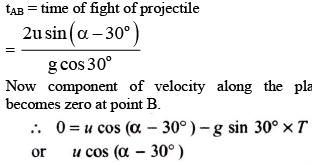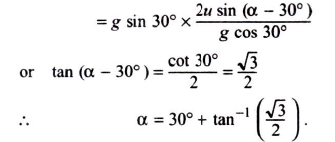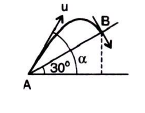QUESTION: 16

With what minimum speed a particle be projected from origin so that it is able to pass through a given point (30 m, 40 m)?

Solution: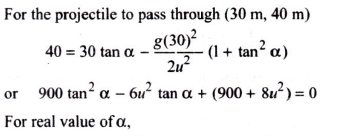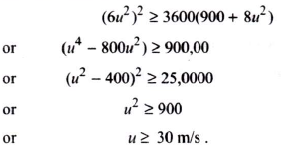QUESTION: 17

A particle is moving along a circular path. The angular velocity, linear velocity, angular acceleration and centripetal acceleration of the particle at any instant respectively are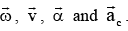. Which of the following relations is not correct?

Solution: Linear velocity is the tangential velocity angular velocity acts in the same direction. So they can never be perpendicular.
QUESTION: 18

A particle is moving along a circular path with uniform speed. Through what angle does its angular velocity change when it completes half of the circular path?

Solution:

Angular velocity is always directed perpendicular to the plane of the circular path. Hence, required change in angle = 0o.

QUESTION: 19

A wheel is subjected to uniform angular acceleration about its axis. Initially its angular velocity is zero. In the first 2 sec, it rotates through an angle q1; in the next 2 sec it rotates through an additional angle θ2, the ratio θ21 is

Solution: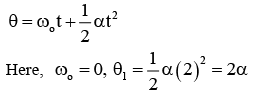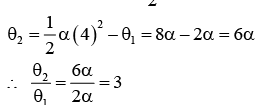QUESTION: 20

A car is moving along a circular path of radius 500 m with a speed of 30 m/s. If at some instant, its speed increases at the rate of 2 m/s2, then at that instant the magnitude of resultant acceleration will be

Solution:

Centripetal acceleration,  ac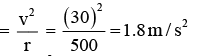Tangential acceleration,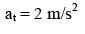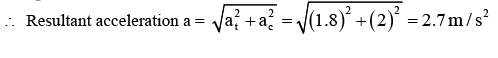QUESTION: 21

If the equation for the displacement of a particle moving on a circular path is given by  θ  = 2t3 + 0.5 where θ is in radian and t in second, then the angular velocity of the particle at  t = 2 s is

Solution: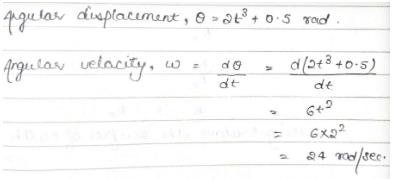QUESTION: 22

Which of the following statements is false for a particle moving in a circle with a constant angular speed?

Solution:

For a particle moving in a circle with constant angular speed, the velocity vector is always tangent to the circle and the acceleration vector always points towards the centre of the circle or is always along the radius of the circle. Since, the tangential vector is perpendicular to radial vector, therefore, velocity vector will be perpendicular to the acceleration vector. But in no case acceleration vector is tangent to the circle.

QUESTION: 23

Which motion does not require force to maintain it?

Solution:

Uniform straight line motion is a type of linear motion. It works according to Newton's First law of motion i.e. objects that do not experience any net force will continue to move in a straight line with a constant velocity until they are subjected to a net force.

QUESTION: 24

A point P moves in counter clockwise direction on a circular direction on a circular path shown in the adjoining figure. The movement of P is such that it sweeps out a length s = t3 + 5, where s is in meter and ‘t’ is in second. The radius of the path is 20 m. The acceleration of P when t = 2 s is nearly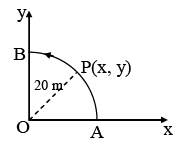Solution: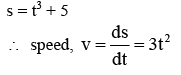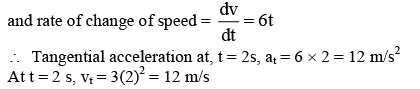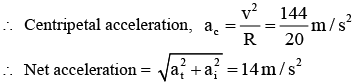QUESTION: 25

Two trains are each 50 m long moving parallel to each other at speeds 10 m/s and 15 m/s respectively, at what time will they pass each other?

Solution:

As both the trains are moving in the opposite direction, the relative speed of each train with respect to each other be, v=10+15=25m/s
Here distance covered by each train = sum of their lengths =50+50=100m
∴ Required time=100/25​=4sec

QUESTION: 26

Two cars are moving in same direction with speed of 30 kmph. They are separated by a distance of 5 km. What is the speed of a car moving in opposite direction if it meets the two cars at an interval of 4 min?

Solution:

Lets say the speed of second car be v. Now with respect to car 1, speed of car 2 is v - 30. Now if the cross each other at just after 4 min then the distance traveled by car 2 relative to car 1 is (v -30) x 4/60 and we know the distance travelled relatively is 5km
Thus we get
(v -30) x 4/60 = 5
V = 300/4 - 30
= 45 km/h

QUESTION: 27

Two particles start simultaneously from the same point and move along two straight liens. One with uniform velocity v and other with a uniform acceleration a. If a is the angle between the lines of motion of two particles then the least value of relative velocity will be at time given by

Solution:

vr is subtraction of vectors. Hence,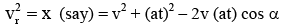Now, vr will be minimum when x is minimum.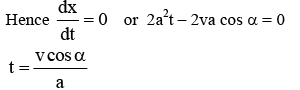QUESTION: 28

A man can row a boat in still water with a velocity of 8 kmph. Water is flowing in a river with a velocity of 4 kmph. At what angle should he row the boat so as to reach the exact opposite point?

Solution:
QUESTION: 29

A man can swim in still water at a speed of 6 kmph and he has to cross the river and reach just opposite point on the other bank. If the river is flowing at a speed of 3 kmph, and the width of the river is 2 km, the time taken to cross the river is (in hours)

Solution:

To reach the exact opposite end the parallel component of velocity would nullify the velocity of the river, hence we get 6.sin a = 3 , where a is the angle with perpendicular to river flow.
Thus we get sin a = ½ and cos a =√3/2
Thus the perpendicular component of velocity, 6.cos a =  3√3
Thus time taken is 2 / 3√3

QUESTION: 30

A person swims at 135o to current of river, to meet target on reaching opposite point. The ratio of person’s velocity to river water velocity is

Solution:

Vr = Vp sin45
Vr = Vp 1/√2
Vr/Vp = 1/√2
Vp/Vr = √2